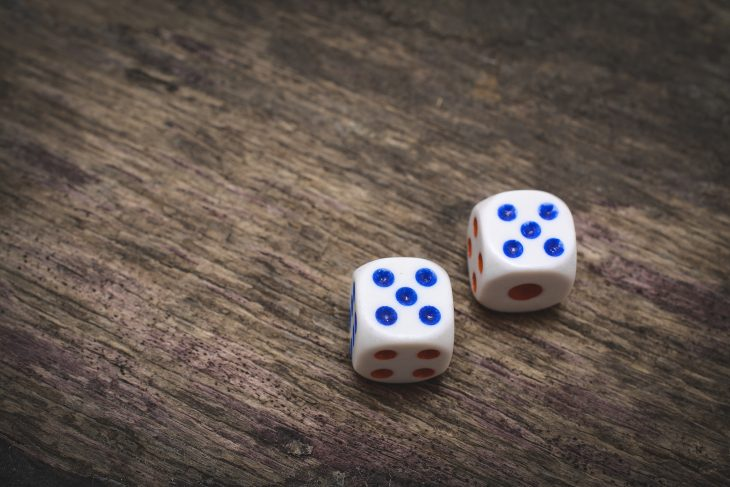# Doubles FactsSunny

Published: 16 May 2023Math can often be intimidating, but it doesn’t have to be! Learning certain facts and equations can make solving complicated problems simpler, faster, and even spark an interest in mathematics. Today we’re going to focus on a fundamental facet of addition – doubles – with some fun facts that will help you boost your math knowledge! So buckle up as we explore 10 entertaining doubles facts that are sure to give your brain a workout.

## The Power of Doubling

Doubles are more than just simple addition problems; they can also be a gateway to understanding the concept of exponents. For example, if you know that 2 + 2 = 4, you can easily grasp that 2 raised to the power of 2 (2^2) equals 4. This understanding of exponents can be extended to other numbers as well.

## Breaking Down Larger Problems

Mastering doubles can help you break down more significant addition problems into smaller, more manageable chunks. For instance, if you need to add 8 + 6, you can break it down into 8 + 2 + 4, or even 6 + 6 – 2, leveraging your knowledge of doubles facts to solve the problem more quickly.

Doubles can also make multiplication problems easier to solve. For example, knowing that 5 + 5 = 10 can help you understand that 5 x 2 = 10. This connection between doubles and multiplication can be especially useful when teaching younger students the fundamentals of multiplication.

## Doubling and Halving

Understanding doubles can also help with division problems. If you know that 6 + 6 = 12, you can quickly deduce that half of 12 is 6. This technique of halving and doubling can be applied to more complex problems as well, making it easier to perform mental calculations.

## Odd and Even Numbers

Doubles can help you recognize the difference between odd and even numbers. All doubles result in even numbers since the sum of two equal numbers will always be divisible by 2. This understanding can be helpful when solving more complex math problems that involve identifying odd or even numbers.

## The Relationship with Near Doubles

Once you have mastered doubles, you can use them to solve near doubles problems. Near doubles are addition problems where the two numbers are one unit apart (e.g., 3 + 4, 7 + 8). For example, if you know that 5 + 5 = 10, you can quickly figure out that 5 + 6 = 11 by adding one to the doubles.

## Squares and Square Roots

Doubles can be a stepping stone to understanding squares and square roots. A square is the result of a number multiplied by itself (e.g., 4^2 = 4 x 4 = 16). Conversely, a square root is a number that, when multiplied by itself, results in a given number (e.g., the square root of 16 is 4). By knowing your doubles facts, you can more easily recognize the relationship between squares and square roots.

## Real-World Applications

Doubles can be useful in everyday situations, such as calculating tips, dividing portions, or estimating distances. For example, if you want to leave a 20% tip on a \$30 bill, you can use the doubles fact 30 + 30 = 60 to quickly calculate that 10% of the bill is \$3, and then double it to find that a 20% tip would be \$6. This practical application of doubles can make everyday tasks simpler and faster.

## Building a Strong Foundation for Higher Mathematics

Having a solid grasp of doubles facts can set the foundation for more advanced mathematical concepts, such as algebra, geometry, and calculus. By understanding these basic facts, you will be better prepared to tackle more complex mathematical problems with confidence and ease.

## Improving Mental Math Skills and Confidence

Finally, mastering doubles facts can significantly improve your mental math skills and boost your confidence in your mathematical abilities. By knowing these facts and being able to apply them to various problems, you will feel more at ease when faced with mathematical challenges.

## Conclusion

Doubles have a lot to offer when it comes to learning math, from the convenience and familiarity of the doubling facts and equations to the realization of how to use them. There isn’t always one single way of getting an answer, which allows us to strengthen our problem-solving skills. Being comfortable with doubles can be a great help in developing confidence in complex math topics, particularly addition. Even if it’s been a while since you’ve worked on any kind of arithmetic, doubles can be approached as an adventure connecting numbers and stimulating your mind! With these 10 amazing facts about doubles in your arsenal, rising above intimidating mathematical challenges no longer has to seem impossible. Ready set double….it’s time for action!